# Experimental study on electrostatic atomization effect of capillary-plate electrode

## Jianhe Liu1, Changqing Sun2

1, 2Advanced Manufacturing Research Laboratory, Changchun University of Science and Technology, Changchun, China

1Corresponding author

Vibroengineering PROCEDIA, Vol. 48, 2023, p. 100-106. https://doi.org/10.21595/vp.2022.23087
Received 29 November 2022; received in revised form 13 December 2022; accepted 15 December 2022; published 11 February 2023

62nd International Conference on Vibroengineering in Almaty, Kazakhstan, February 10-11, 2023

Copyright © 2023 Jianhe Liu, et al. This is an open access article distributed under the Creative Commons Attribution License, which permits unrestricted use, distribution, and reproduction in any medium, provided the original work is properly cited.
Views 18
CrossRef Citations 0
Abstract.

The electrostatic atomization effect of liquid in high voltage electrostatic field was studied under capillary-flat electrodes. The effects of charging voltage, liquid flow rate, and the distance between a capillary and flat electrode on droplet size distribution were analyzed by the DP-02 laser particle size analyzer. The single-factor method was used to analyze the effects. The experimental results show that the charging voltage, the liquid flow rate, and the distance between the capillary and the plate electrode greatly influence the droplet's atomization effect. The median particle size D50 and the distribution span (Span) decrease with the increase of charging voltage, the reduction of liquid flow and the decline of the distance between capillary and plate electrode. That is, the droplet particle size distribution is better, and thus the droplet electrostatic atomization effect is better.

Highlights
• The effect of different factors on liquid atomization in high voltage electrostatic field under capillary and plate electrodes was studied.
• The effects of charging voltage, liquid flow rate and distance between capillary and plate electrode on droplet size distribution were analyzed by laser particle size analyzer.
• The median particle size D50 and distributed span (Span) were used to measure the liquid atomization effect.
• The more uniform the droplet size distribution, the better the electrostatic atomization effect.

Keywords: capillary-plate electrode, electrostatic atomization, Particle size distribution, laser particle size analyzer.

#### 1. Introduction

When the liquid flows out of the charged capillary at a certain flow rate, the surface of the liquid will generate electric field force along the surface under the action of an electric field. When the electric field force is large enough, dispersed phase droplets or jets will be generated at the end of the capillary. This process is called capillary electrostatic atomization [1-2]. Electrostatic atomization  is a process in which liquid is broken into small charged droplets under electrostatic force. Compared with other atomization methods, atomization with this kind of static electricity has its unique advantages: for example, the droplet particle size is smaller, up to nanometers; The droplet distribution is more uniform, and the nanodispersion is strong. The atomized flow field is highly controllable due to the electric field. High deposition efficiency, etc., so it is widely used in spraying, dust removal , film preparation , pesticide spraying , and other fields. The process of electrostatic atomization is influenced by many factors, such as charging voltage, liquid flow rate, and the distance between the capillary and flat electrode, etc., and the atomization process presents diversity .

In this paper, the effects of charging voltage, liquid flow rate and the distance between a capillary and flat electrode on the droplet size distribution were compared and analyzed. The influences of various factors on the electrostatic atomization effect were revealed, which provided a necessary basis and guidance for improving and developing the electrostatic atomization system in different situations.

#### 2.1. The mechanism of charged droplet breaking

When the droplet is suspended and stationary, a pressure difference ${P}_{\sigma }$ is generated inside and outside the droplet. When charged, an expansive force ${P}_{e}$ will be developed in the droplet. The force model of a charged droplet is shown in Fig. 1: surface tension $\sigma$ and ${\sigma }_{1}$ electrostatic force f and ${f}_{1}$ are two pairs of forces and reaction forces, respectively. According to the theory of electrostatic field, at any point E outside the charged droplet, as shown in Fig. 2:

(1)
$E=\frac{q}{4\pi {\epsilon }_{0}{r}^{2}},$

where $q$ is the charge of the droplet, C; ${\epsilon }_{0}$ is the vacuum dielectric constant, F/m; $r$ is the distance from the droplet at any point, mm.

Fig. 1. Droplet stress model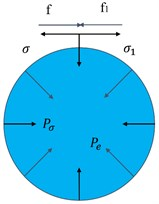Fig. 2. Droplet electric field model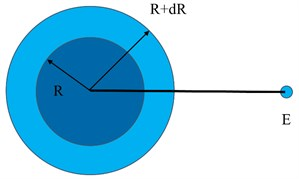The droplet surface potential $V$ and the electric energy stored on the droplet $W$ are respectively:

(2)
$V=-{\int }_{\infty }^{R}Edr=\frac{q}{4\pi {\epsilon }_{0}R},$
(3)
$W=-{\int }_{0}^{q}Vdq=\frac{{q}^{2}}{8\pi {\epsilon }_{0}R}.$

With $q$ unchanged, if the droplet radius increases from $R$ to $R+dR$, we can get:

(4)
$dW=-\frac{{q}^{2}}{8\pi {\epsilon }_{0}{R}^{2}}dR.$

The work done by ${P}_{e}$ in this process should be equal to $dE$, then:

(5)
$dW=-4\pi {R}^{2}{P}_{e}dR.$

Make the above two formulas equal, and you can get:

(6)
${P}_{e}=\frac{{q}^{2}}{32\pi {\epsilon }_{0}{R}^{2}}.$

When ${P}_{e}={P}_{\sigma }$, the charged droplet reaches the critical breaking state, and then:

(7)
${q}_{max}=8\pi \sqrt{{\epsilon }_{0}\sigma {R}^{3/2}},$

where ${q}_{max}$ is charged limit of the droplet, C; $V$ is potential, V; $W$ is electric energy, J.

When the surface of the droplet is charged, the surface tension of the droplet decreases under the influence of electrostatic force, and the surface tension of the droplet decreases to:

(8)
${\sigma }_{1}=\sigma -\frac{{q}^{2}}{64{\pi }^{2}{\epsilon }_{0}{R}^{3}}.$

Therefore, the actual charge when the droplet breaks is less than.

#### 2.2. The evaluation index of atomization effect

Droplet size distribution is a critical evaluation index of the atomization effect. In the measurement statistics, the median particle size D50 is selected as the atomized droplet size measurement index. The size distribution span is used to measure the size distribution width, expressed in equation 9. The smaller the values of median particle size D50 and span (Span) indicate that the overall particle size of the droplet is smaller, and the particle size is more concentrated, so the liquid atomization effect is better:

(9)
$Span=\frac{{D}_{90}-{D}_{10}}{{D}_{50}}.$

#### 2.3. Experimental apparatus and method

Fig. 3 is a schematic diagram of an electrostatic atomization device. To study the effect of external parameters on electrostatic atomization, a laser particle size analyzer measured the particle size distribution of atomized droplets.

The measuring area of the laser particle size analyzer (DP-02) is shown in Fig. 4. The distance h from the bottom of the capillary is variable. The particle size and distribution of droplets in the measuring area are measured and analyzed through the light scattering principle.

Fig. 3. Droplet size distribution measuring device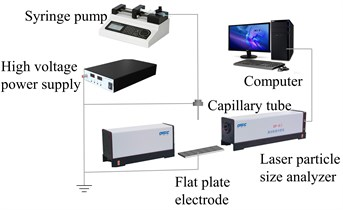Fig. 4. Laser particle size analyzer measurement area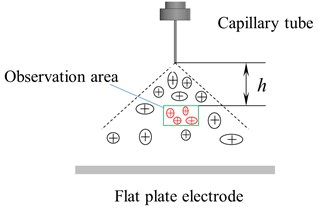Table 1. Physical property constants of liquids

 Liquor / (kg∙m-3) $\mu$ / (10-3 Pa∙s) $\gamma$/ (10-3 S∙m-1) $\sigma$ / (10-3 N∙m-1) Water 998 1.007 0.200 73.2

#### 3.1. Influence of charging voltage on atomization effect

Under the condition of the liquid flow of 80 μl/min and spacing between a capillary and flat electrode of 80 mm, the relation curve between charging voltage, median particle size D50, and distribution span (Span) is shown in Fig. 5. As can be seen in Fig. 5, the median particle size D50 and distribution span (Span) of atomized droplets both decrease with the increase of charging voltage. This is because the increase of charging voltage will strengthen the electric field intensity between the capillary and the plate electrode. Thus, the atomized droplet size is reduced, and the distribution becomes more uniform, which can achieve better atomization effect.

Fig. 5. The relation curve between charging voltage and particle size distribution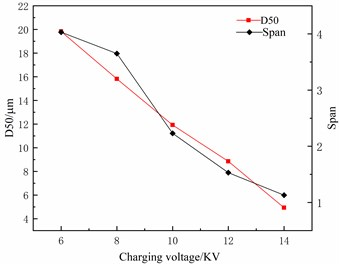Fig. 6 shows the distribution of aqueous particles and the statistical distribution of particle size under 14 kV voltage. It can be seen from Fig. 6 that the droplet distribution is relatively uniform, and the median particle size is about 9 μm.

Fig. 6. Fog drops distribution of aqueous solution at 14 kV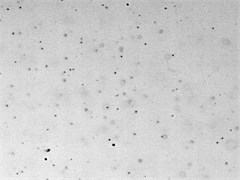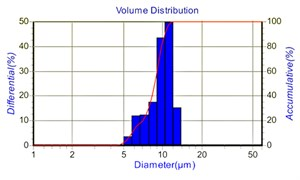#### 3.2. Influence of liquid flow rate on atomization effect

Under the condition that the charging voltage is 10 KV and the distance between the capillary and the plate electrode is 80 mm, the relation curve of the liquid flow with the median particle size D50 and the distribution span (Span) is shown in Fig. 7. As seen in Fig. 7, the median particle size D50 and distribution span of atomized droplets increases with the increase of liquid flow rate. This is because, with the increase in liquid flow rate, some particles float away before they arrive or atomize, thus increasing the particle size and distribution span of droplets, indicating that liquid flow rate should be minimized in the electrostatic atomization system.

Fig. 7. The relationship between liquid flow and particle size distribution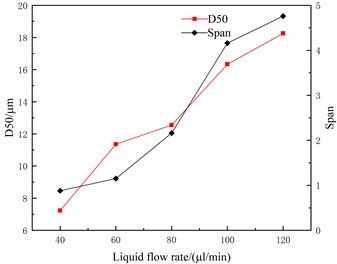Fig. 8 shows the distribution of aqueous droplets at two different liquid flows. By comparison, it is found that the droplet particle size and distribution range are significantly more extensive than 40 μl/min, at 80 μl/min. The number of droplet particles in the same region is minimal because the droplet distribution range is relatively wide at 80 μl/min. So the number of droplets displayed in the same area will be relatively small.

Fig. 8. Distribution of aqueous droplets at different liquid flows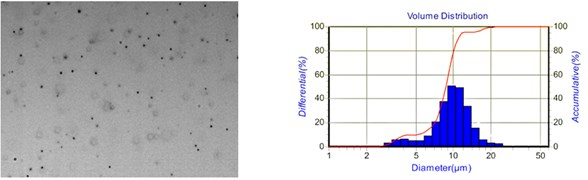a) 40 μl/min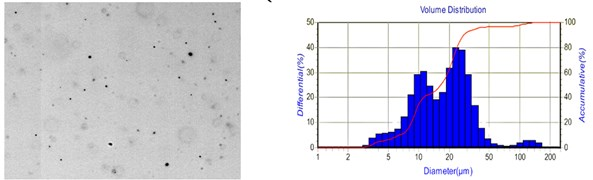b) 80 μl/min

#### 3.3. Effect of capillary plate electrode spacing on atomization effect

Under the charging voltage of 10 KV and liquid flow of 80 μl/min, the relation curves of capillary-plate electrode spacing with median particle size D50 and distribution span (Span) are shown in Fig. 9. As seen in Fig. 9, the median particle size D50 and the distribution span (Span) of atomized droplets increase with the capillary-flat electrode spacing. This is because the increase of the capillary-flat electrode spacing reduces the electric field intensity in the atomized region, thus worsening the atomization effect of droplets.

Fig. 9. The relationship between capillary-plate electrode spacing and particle size distribution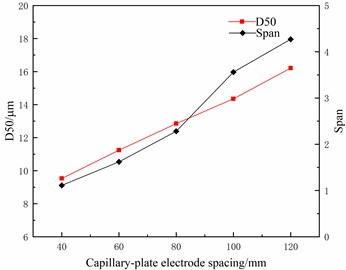Fig. 10 shows the droplet distribution diagram of the aqueous solution under two different spacings. By comparison, it is evident that the larger the spacing, the larger the droplet size and the more comprehensive the droplet size distribution range. Therefore, appropriately reducing the capillary-plate electrode spacing helps improve the droplet atomization effect.

Fig. 10. Droplet distribution of aqueous solution at different spacing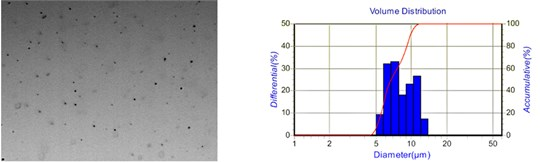a) 40 mm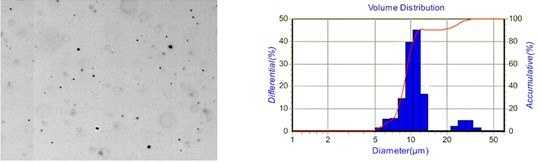b) 60 mm

#### 4. Conclusions

In this paper, the electrostatic atomization effect of liquid in high voltage electrostatic field was experimentally studied under capillary-plate induction electrode, and the effects of charging voltage, liquid flow rate, and the distance between capillary and plate electrode on droplet size distribution were compared and analyzed. The following conclusions were drawn:

1) Increasing the charging voltage will increase the electric field intensity between the capillary and the plate electrode, making the atomized droplets smaller in size, more uniform in distribution and better in atomization effect.

2) The increase of liquid flow will increase the number of liquid particles simultaneously, and some particles will drift away before being atomized and broken so that the particle size and distribution span of the droplets will increase, and the atomization effect will become worse.

3) The increase of the spacing between capillary and plate electrodes will reduce the intensity of the field in the atomization region, which will make the atomization effect worse.

#### Acknowledgements

The authors would like to thank the Science and technology development plan project of Jilin province No. 20190304132YY.

1. W. Zhentao et al., “Experiment on electro-spraying modes with double-capillaries,” Journal of Jiangsu University (Natural Science Edition), Vol. 39, No. 3, pp. 284–289, 2018, https://doi.org/10.3969/j.issn.1671-7775.2018.03.007 [Search CrossRef]
2. Q. Wang, Z. Wang, Y. Jiang, and S. Yang, “Experimental study of electro-spraying modes of deionized water in atmospheric environment,” Experimental and Computational Multiphase Flow, Vol. 3, No. 1, pp. 38–46, Mar. 2021, https://doi.org/10.1007/s42757-019-0057-3 [Publisher]
3. W. Junfeng et al., “Effects of liquid properties on electrostatic spray characteristics,” Journal of Drainage and Irrigation Machinery Engineering, Vol. 30, No. 4, pp. 469–472, 2012, https://doi.org/10.3969/j.issn.1674-8530.2012.04.018 [Search CrossRef]
4. D. Quanlong et al., “Numerical simulation on the influence of wire and plate arrangement on electrostatic precipitator performance,” Journal of Environmental Engineering, pp. 1–16, 2022, https://doi.org/10.12030/j.cjee.202206057 [Search CrossRef]
5. Z. Chenjie, C. Haiquan, and Y. Youhai, “Preparation of electrochromic film by combination of electrostatic spray method/in-situ washing method,” Journal of Materials Engineering, Vol. 50, No. 1, pp. 109–116, 2022, https://doi.org/10.11868/j.issn.1001-4381.2020.001087 [Search CrossRef]
6. L. Xingguang, L. Jianhe, and X. Yanming, “Study on application of pesticide spraying by electrostatic atomization technology,” The Journal of New Industrialization, Vol. 7, No. 9, pp. 53–57, 2017, https://doi.org/10.19335/j.cnki.2095-6649.2017.09.009 [Search CrossRef]
7. Qisi W. et al., “Analysis of evolution process of twin-capillary electro-spraying regime,” Journal of Jiangsu University (Natural science Edition), Vol. 43, No. 1, pp. 38–44, 2022, https://doi.org/10.3969/j.issn.1671-7775.2022.01.006 [Search CrossRef]